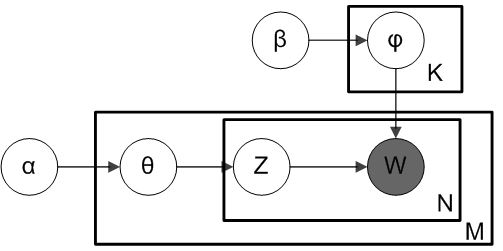# Topic Model-- LDA

#### Introduction

In real Infromation Retrieval (Google, Bing), when we gather many online documents, we need to classify them according to their topic. For this task, we need a machine learning algorithm to do the classification.

One way is to use naive bayes to choose the topic with maximal posterior probability $P(topic |document)$.

In this approach, there are 2 models available: multivariate Bernoulli model and multinomial model.

But in 2003, David Blei, Andrew Ng, and Michael Jordan introduced LDA into this area, presented as a graphical model for topic discovery.

#### Basics

In LDA, each document may be viewed as a mixture of various topics. This is similar to probabilistic latent semantic analysis, except that in LDA the topic distribution is assumed to have a Dirichlet prior. In practice, this results in more reasonable mixtures of topics in a document.

#### ModelThe outer plate represents documents, while the inner plate represents the repeated choice of topics and words within a document. $M$ denotes the number of documents, $N$ the number of words in a document.

$\alpha$ is the parameter of the Dirichlet prior on the per-document topic distribution. $\beta$ is the parameter of the Dirichlet prior on the per-topic word distribution. $\Theta_i$ is the topic distribution for document i. $\phi_k$ is the word distribution for topic k $z_{ij}$ is the topic for the $j$th word in document i $w_{ij}$ is the specific word

The $w_{ij}$ are the only observable variables, and the other variables are latent variables.

The gernerative process behind is that documents are represented as random mixtures over latent topics, where each topic is characterized by a distribution over words. LDA assumes the following generative process for each document $i$ in a corpus D:

1. Choose $\Theta_i \thicksim Dir(\alpha)$, where $i \in {1,\ldots M}$ and $Dir(\alpha)$ is the Dirichlet distribution for parameter $\alpha$

2. Choose $\phi_k \thicksim Dir(\beta)$, where $k \in {1,\ldots,K}$

3. For each of the word $w_{ij}$, where $j \in {1,\ldots,N_i}$ 3a. Choose a topic $z_{ij} \thicksim Multinomial(\Theta_i)$ 3b. Choose a word $w_{ij} \thicksim Multinomial(\phi_{zi,j})$

#### Inference

There is a long long long inference process… So if you are interested in the entire inference, refer to PRML